# pytorch常用损失函数

损失函数的基本用法：

criterion = LossCriterion() #构造函数有自己的参数
loss = criterion(x, y) #调用标准时也有参数

1.BCELoss(二分类)

CLASS torch.nn.BCELoss(weight=None, size_average=None, reduce=None, reduction='mean')

unreduced loss函数(即reduction参数设置为'none')为：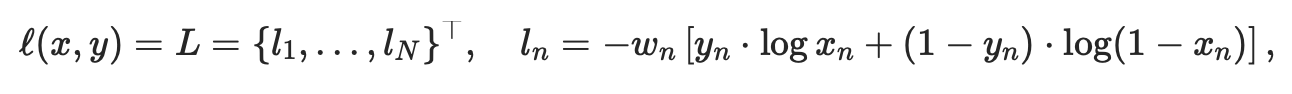N表示batch size，xn为输出，yn为目标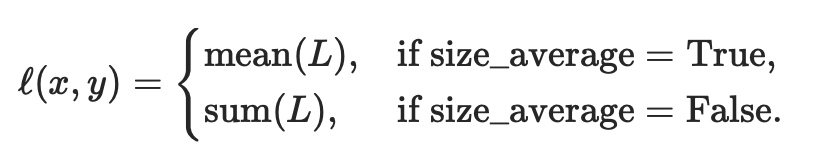这是用来测量误差error的重建，例如一个自动编码器。注意 0<=target[i]<=1。

• weight (Tensor,可选) – 每批元素损失的手工重标权重。如果给定，则必须是一个大小为“nbatch”的张量。
• size_average (bool, 可选) – 弃用(见reduction参数)。默认情况下，设置为True，即对批处理中的每个损失元素进行平均。注意，对于某些损失，每个样本有多个元素。如果字段size_average设置为False，则对每个小批的损失求和。当reduce为False时，该参数被忽略。默认值:True
• reduce (bool,可选) – 弃用(见reduction参数)。默认情况下，设置为True，即根据size_average参数的值决定对每个小批的观察值是进行平均或求和。如果reduce为False，则返回每个批处理元素的损失，不进行平均和求和操作，即忽略size_average参数。默认值:True
• reduction (string,可选) – 指定要应用于输出的reduction操作:' none ' | 'mean' | ' sum '。“none”:表示不进行任何reduction，“mean”:输出的和除以输出中的元素数，即求平均值，“sum”:输出求和。注意:size_average和reduce正在被弃用，与此同时，指定这两个arg中的任何一个都将覆盖reduction参数。默认值:“mean”

形状：

• 输入：(N,*), *代表任意数目附加维度
• 目标：(N,*)，与输入拥有同样的形状
• 输出:标量scalar，即输出一个值。如果reduce为False,即不进行任何处理，则(N,*)，形状与输入相同。

举例：

m = nn.Sigmoid()
loss = nn.BCELoss()
target = torch.empty(3).random_(2)
output = loss(m(input), target)
output.backward()

input,target,output

(tensor([-0.8728,  0.3632, -0.0547], requires_grad=True),
tensor([1., 0., 0.]),
tensor(0.9264, grad_fn=<BinaryCrossEntropyBackward>))

m(input)结果为：

tensor([0.2947, 0.5898, 0.4863])

input.grad

tensor([-0.2351,  0.1966,  0.1621])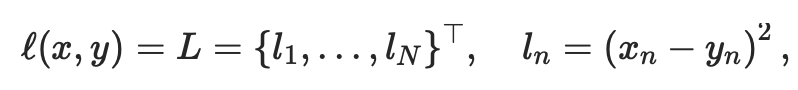• 对w求导： ∂L / ∂w = (xn - yn) * σ'(z) * z= (xn - yn) * σ'(z) * x
• 对b求导：  ∂L / ∂b = (xn - yn) * σ'(z)

• 对w求导： ∂L / ∂w = 1/n *  Σi xn * (σ(z)-yn)
• 对b求导：  ∂L / ∂b =1/n *  Σi (σ(z)-yn)

2.BCEWithLogitsLoss

CLASS torch.nn.BCEWithLogitsLoss(weight=None, size_average=None, reduce=None, reduction='mean', pos_weight=None)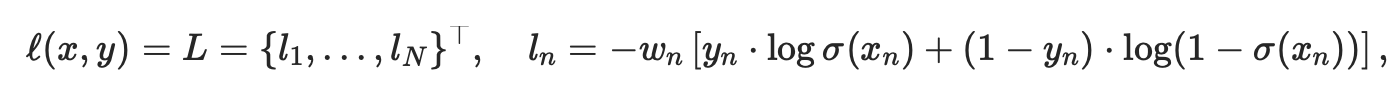• pos_weight (Tensor,可选) –正值例子的权重，必须是有着与分类数目相同的长度的向量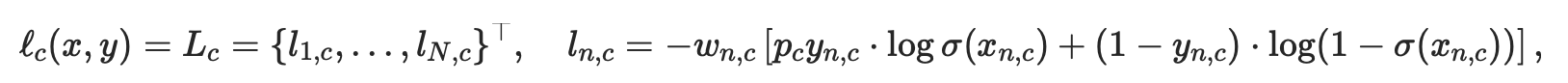c表示类的数量(c>1表明是多标签二进制分类，c=1表明是单标签二进制分类)，n为一批中的例子数量，pc为类别c的正值的权重,解决正负例样本不均衡的情况

pc>1增加召回率，pc<1增加准确性

m = nn.Sigmoid()
loss = nn.BCELoss()
target = torch.empty(3).random_(2)
output = loss(m(input), target)

loss = nn.BCEWithLogitsLoss()
target = torch.empty(3).random_(2)
output = loss(input, target)

4.NLLLoss(多分类)

CLASS torch.nn.NLLLoss(weight=None, size_average=None, ignore_index=-100, reduce=None, reduction='mean')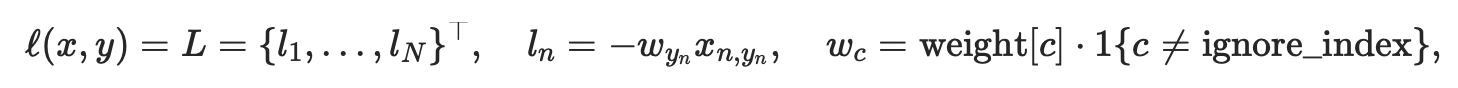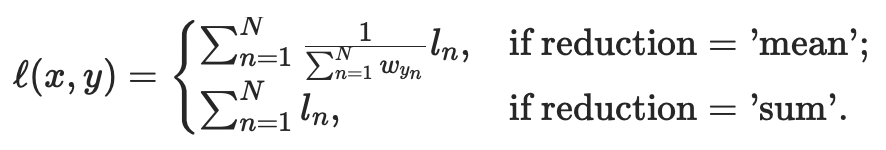• ignore_index (int, optional) – 指定一个被忽略的目标值，该目标值不影响输入梯度。当size_average为真时，对非忽略目标的损失进行平均。

形状：

• 输入：(N,C), C代表类别的数量；或者在计算高维损失函数例子中输入大小为(N,C,d1,d2,...,dK)，k>=1
• 目标：(N)，与输入拥有同样的形状，每一个值大小为为 0≤targets[i]≤C−1 ；或者在计算高维损失函数例子中输入大小为(N,C,d1,d2,...,dK)，k>=1
• 输出:标量scalar。如果reduction='none',则其大小与目标相同，为(N)或(N,C,d1,d2,...,dK)，k>=1

低维举例：

m = nn.LogSoftmax(dim=1)
loss = nn.NLLLoss()
# input is of size N x C = 3 x 5
input

tensor([[-0.8676,  1.5017,  0.2963, -0.9431, -0.0929],
[ 0.3540,  1.0994, -1.1085, -0.4001,  0.0102],
[ 1.3653, -0.3828,  0.6257, -2.4996,  0.1928]], requires_grad=True)

nn.LogSoftmax(dim=1)即先进行Softmax计算(得0-1的值)，再取Log，得到的都是负数：

m(input)

tensor([[-2.8899, -0.5205, -1.7259, -2.9653, -2.1152],
[-1.5082, -0.7628, -2.9707, -2.2623, -1.8520],
[-0.6841, -2.4323, -1.4237, -4.5490, -1.8566]],
grad_fn=<LogSoftmaxBackward>)

#each element in target has to have 0 <= value < C
target = torch.tensor([1,0,4])
output = loss(m(input), target)
output

tensor(1.2951, grad_fn=<NllLossBackward>)

# 2D loss example (used, for example, with image inputs)
N, C = 5,4
loss = nn.NLLLoss()
# input is of size N x channel x height x width
data = torch.randn(N, 16, 10, 10)
conv = nn.Conv2d(16, C, (3, 3))#输出为5*8*8
m = nn.LogSoftmax(dim=1)
# each element in target has to have 0 <= value < C
target = torch.empty(N, 8, 8, dtype=torch.long).random_(0, C) #target.size()=target = torch.empty(N, 8, 8, dtype=torch.long).random_(0, C)
output = loss(m(conv(data)), target)
output

tensor(1.5501, grad_fn=<NllLoss2DBackward>)

5.CrossEntropyLoss(多分类）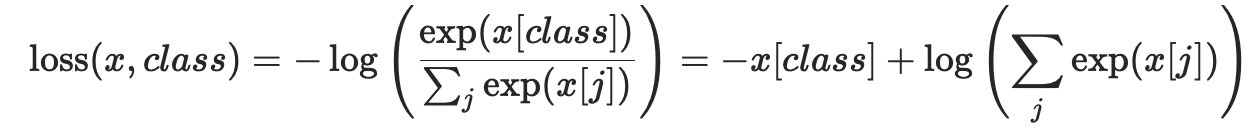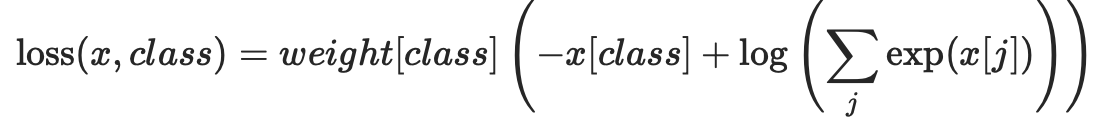该损失函数也能被使用在高维输入中，通过提供大小为

m = nn.LogSoftmax(dim=1)
loss = nn.NLLLoss()
# input is of size N x C = 3 x 5
#each element in target has to have 0 <= value < C
target = target = torch.empty(3, dtype=torch.long).random_(5)
output = loss(m(input), target)

loss = nn.CrossEntropyLoss()
# input is of size N x C = 3 x 5
#each element in target has to have 0 <= value < C
target = target = torch.empty(3, dtype=torch.long).random_(5)
output = loss(input, target)

6.L1Loss(L1 norm)

CLASS torch.nn.L1Loss(size_average=None, reduce=None, reduction='mean')

unreduced loss函数(即reduction参数设置为'none')为：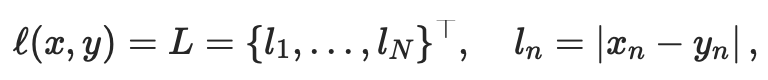N表示batch size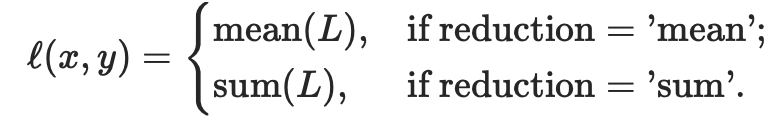x和y是有着n个向量的任意形状的张量

mean先对所有元素进行sum操作,然后除以n

形状：

• 输入：(N,*), *代表任意数目附加维度
• 目标：(N,*)，与输入拥有同样的形状
• 输出:标量scalar，即输出一个值。如果reduction='none',即不进行任何处理，则为(N,*)，形状与输入相同。

举例：

loss = nn.L1Loss()
target = torch.randn(1, 2)#tensor([[0.6789, 0.9831]])
output = loss(input, target)#tensor(1.9424, grad_fn=<L1LossBackward>)

output= (|-0.0625-0.6789| + |-2.1603-0.9831|) / 2 = 1.9424

7.MSELoss(L2 norm)

unreduced loss函数(即reduction参数设置为'none')为：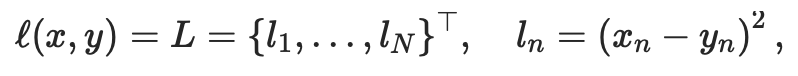N表示batch size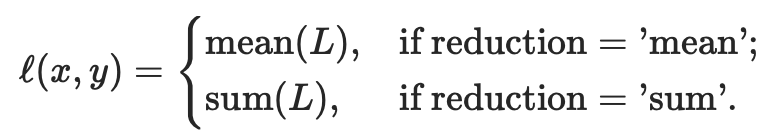x和y是有着n个向量的任意形状的张量

mean先对所有元素进行sum操作,然后除以n

形状：

• 输入：(N,*), *代表任意数目附加维度
• 目标：(N,*)，与输入拥有同样的形状

loss = nn.MSELoss()
target = torch.randn(1, 2)#tensor([[ 0.7117, -0.1200]])
output = loss(input, target)#tensor(5.1303, grad_fn=<MseLossBackward>)

output =( (-1.4445-0.7117)2 + ( -2.4888 + 0.1200 )2 ) / 2 = 5.1303

8.SmoothL1Loss

CLASS torch.nn.SmoothL1Loss(size_average=None, reduce=None, reduction='mean')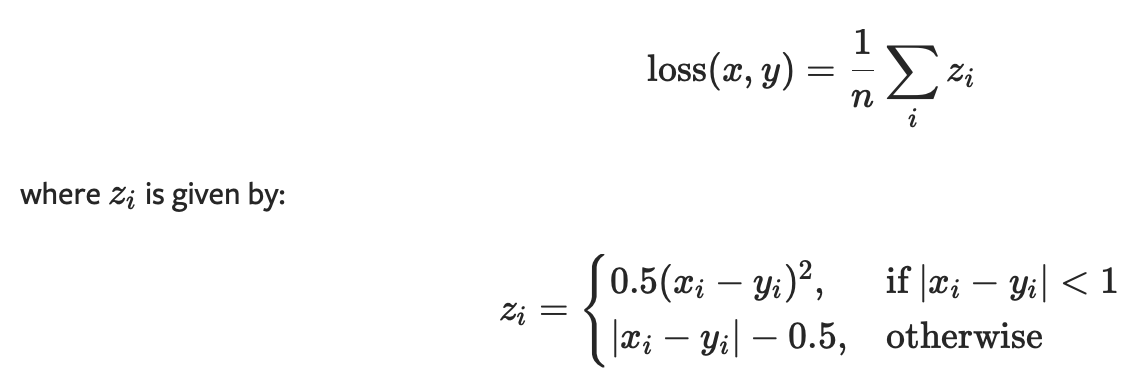x和y是有着n个向量的任意形状的张量

mean先对所有元素进行sum操作,然后除以n

形状：

• 输入：(N,*), *代表任意数目附加维度
• 目标：(N,*)，与输入拥有同样的形状
• 输出:标量scalar，即输出一个值。如果reduction='none',即不进行任何处理，则为(N,*)，形状与输入相同。

posted @ 2019-05-14 18:06  慢行厚积  阅读(59061)  评论(3编辑  收藏  举报